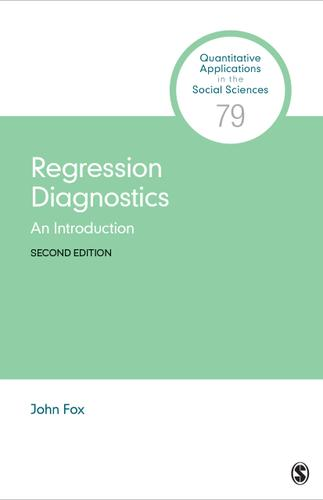# Regression Diagnostics

2nd Edition

An Introduction

John Fox
eISBN-13: 9781544375205## eBook Features

Rent or Buy from \$ 16.00 USD
Note: We do not guarantee supplemental material with textbooks (e.g. CD's, Music, DVD's, Access Code, or Lab Manuals)

## Additional Book Details

Regression diagnostics are methods for determining whether a regression model that has been fit to data adequately represents the structure of the data. For example, if the model assumes a linear (straight-line) relationship between the response and an explanatory variable, is the assumption of linearity warranted? Regression diagnostics not only reveal deficiencies in a regression model that has been fit to data but in many instances may suggest how the model can be improved. The Second Edition of this bestselling volume by John Fox considers two important classes of regression models: the normal linear regression model (LM), in which the response variable is quantitative and assumed to have a normal distribution conditional on the values of the explanatory variables; and generalized linear models (GLMs) in which the conditional distribution of the response variable is a member of an exponential family. R code and data sets for examples within the text can be found on an accompanying website.

ISBNs 9781544375229, 9781544375229, 9781544375205, 1544375204 English 168 2nd